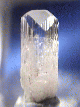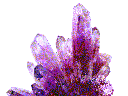DANBURITE
Symmetry
Isometric | Tetragonal | Hexagonal | Trigonal | Monoclinic | Triclinic | Amorphous

# THE ORTHORHOMBIC SYSTEM<><><><><><><><><><><><><><><>

SOME ORTHORHOMBIC
MINERALS

CHRYSOBERYL

OLIVINE
variety: Peridot

The orthorhombic system is based on three unequal axes all at right angles to each other. As can be imagined, as one views down every one of the axes, two unequal axes crossed at right angles can be seen. A possible two fold rotational symmetry is seen in the axes as well as two possible mirror planes that are parallel to the axes.

The orthorhombic system has three classes, with the most symmetrical class having the second largest assortment of minerals represented. As stated above, all axes of the orthorhombic system can serve as two fold rotational axes. They as well can serve as the linear intersection of two perpendicular mirror planes. If all three perpendicular mirror planes are present, then the three crystallographic axes are defined by the intersection of the mirrors. All of this symmetry produces a center of symmetry (an inversion operation). This describes the highest symmetry of the orthorhombic system, Orthorhombic Dipyramidal, with symbology of 2/m 2/m 2/m. This class has, as a gross simplification, a model type form that is a simple rectangular box whose six faces are paired into three sets of different sized rectangles.

The next class, The Orthorhombic Disphenoidal Class, has lost its mirror planes but still has the 3 two fold axes. All the axes are of course perpendicular to each other. The model type crystal is called a disphenoid and is similar to the tetragonal disphenoid of the 22nd and the 24th classes and the tetrahedron of the 31st class. The orthorhombic disphenoid has scalene triangular faces as opposed to the isosceles triangles of the tetragonal disphenoid and the equalateral triangles of the isometric tetrahedron. All these forms appear as opposing wedges.

The final class, Orthorhombic Pyramidal, only has two mirror planes and one two fold axis. The two fold axis serves as the intersection of the mirror planes. Since this class lacks the perpendicular mirror plane or other two fold rotations to the only two fold axis, it can then produce hemimorphic crystals that have a different top from their bottom.

## Orthorhombic Disphenoidal

• Class: 7th
• Symmetry: 2 2 2
• Symmetry Elements: There are only three axes of two fold rotation.
• Axes: None are equal
• Angles: All three angles = 90 degrees.
• Of Note: Crystals can be left or right handed, enantiomorphic.
• Common Forms: The orthorhombic disphenoid, orthorhombic prism and multiple pinacoids.
• Most Common Minerals Known to this Class: Epsomite

## Orthorhombic Pyramidal

• Class: 6th
• Symmetry: 2 m m
• Symmetry Elements: There is only one axes of two fold rotation and there are two mirrors.
• Axes: None are equal
• Angles: All three angles = 90 degrees.
• Of Note: Crystals are hemimorphic or polar.
• Common Forms: The pyramid, prism, dome and pedion.
• Most Common Minerals Known to this Class: Hemimorphite, bertrandite, enargite, natrolite and prehnite.

Isometric | Tetragonal | Hexagonal | Trigonal | Monoclinic | Triclinic | AmorphousAmethyst Galleries'Mineral Gallery MINERALS Groupings Birthstones Gemstones By Name Framed Unframed By Class Carbonates Elements Halides Oxides Phosphates Silicates Sulfates Sulfides Fluorescent Minerals Properties Rocks Search Books